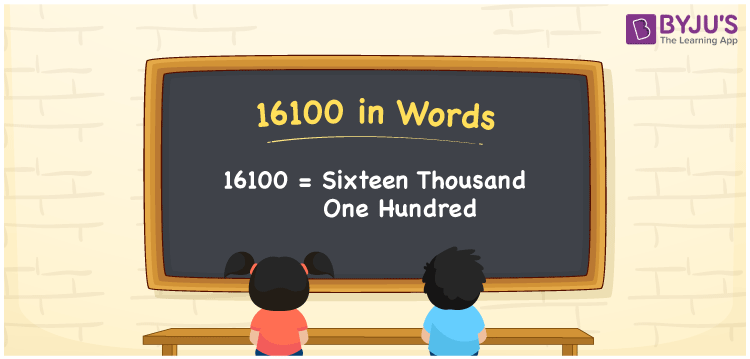# 16100 in words

16100 in words is written as Sixteen Thousand One Hundred. In 16100, 1 has a place value of ten thousand, 6 is in the place value of thousand and 1 has the place value of hundred. The article on Place Value gives more information. The number 16100 is used in expressions that relate to money, distance, social media views, and many more. For example, “Do you have rupees Sixteen Thousand One Hundred in cash”.

 16100 in words Sixteen Thousand One Hundred Sixteen Thousand One Hundred in Numbers 16100

## 16100 in English Words## How to Write 16100 in Words?

We can convert 16100 to words using a place value chart. The number 16100 has 5 digits, so let’s make a chart that shows the place value up to 5 digits.

 Ten thousand Thousands Hundreds Tens Ones 1 6 1 0 0

Thus, we can write the expanded form as:

1 × Ten thousand + 6 × Thousand + 1 × Hundred + 0 × Ten + 0 × One

= 1 × 10000 + 6 × 1000 + 1 × 100 + 0 × 10 + 0 × 1

= 16100.

= Sixteen Thousand One Hundred.

16100 is the natural number that is succeeded by 16099 and preceded by 16101.

16100 in words – Sixteen Thousand One Hundred.

Is 16100 an odd number? – No.

Is 16100 an even number? – Yes.

Is 16100 a perfect square number? – No.

Is 16100 a perfect cube number? – No.

Is 16100 a prime number? – No.

Is 16100 a composite number? – Yes.

## Solved Example

1. Write the number 16100 in expanded form

Solution: 1 x 10000 + 6 x 1000 + 1 x 100 + 0 x 10 + 0 x 1

Or Just 1 x 10000 + 6 x 1000 + 1 x 100

We can write 16100 = 10000 + 6000 + 100 + 00 + 0

= 1 x 10000 + 6 x 1000 + 1 x 100 + 0 x 10 + 0 x 1.

## Frequently Asked Questions on 16100 in words

Q1

### How to write the number 16100 in words?

16100 in words is written as Sixteen Thousand One Hundred.
Q2

### Is 16100 divisible by 3?

No. 16100 is not divisible by 3.
Q3

### Is 16100 divisible by 10?

Yes. 16100 is divisible by 10.